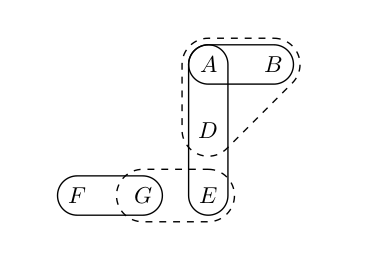# Drawing Hypergraph using TikZ

You can change the inner sep of hyper edge or conditional edge so that they both will have different sizes.

I don't have a good solution for the second part using fit library. I just drew that conditional edge.\documentclass{article}
\usepackage{tikz}
\usetikzlibrary{calc, positioning, fit, shapes.misc}

\begin{document}
\begin{tikzpicture}
[
he/.style={draw, rounded corners,inner sep=0pt},        % he = hyper edge
ce/.style={draw,dashed, rounded corners=10pt}, % ce = condition edge
]

\node (f) at (0,0) {$F$};
\node (g) at (1,0) {$G$};
\node (e) at (2,0) {$E$};
\node (d) at (2,1) {$D$};
\node (a) at (2,2) {$A$};
\node (b) at (3,2) {$B$};

\node [he, fit=(f) (g)] {};
\node [ce, fit=(g) (e)] {};
\node [he, fit=(a) (d) (e)] {};
\node [fit=(a) (b) (d)] (fd){};
\draw [dashed,rounded corners=10pt] ($(fd.south west)+(0,-0.5)$) -- (fd.north west) -- ($(fd.north east)+(0.5,0)$)--cycle;
\end{tikzpicture}
\end{document}


You can use my (semi) library (https://gitlab.com/gruenwald/tikz-hypergraphs) that I made for exactly this purpose:

\documentclass{article}
\usepackage{tikz}
\usepackage{ifthen}             % For tikz-hypergraph
\usetikzlibrary{calc}           % For tikz-hypergraph
\input{tikz-hypergraph}

\begin{document}
\begin{tikzpicture}
[
he/.style={draw, semithick},        % he = hyper edge
ce/.style={draw, dashed, semithick}, % ce = condition edge
]

\node (f) at (0,0) {$F$};
\node (g) at (1,0) {$G$};
\node (e) at (2,0) {$E$};
\node (d) at (2,1) {$D$};
\node (a) at (2,2) {$A$};
\node (b) at (3,2) {$B$};

\draw[he] \hedgeii{a}{b}{3mm};
\draw[he] \hedgeii{a}{e}{3mm};
\draw[ce] \hedgeiii{a}{b}{d}{4mm};
\draw[ce] \hedgeii{g}{e}{4mm};
\draw[he] \hedgeii{f}{g}{3mm};

\end{tikzpicture}
\end{document}